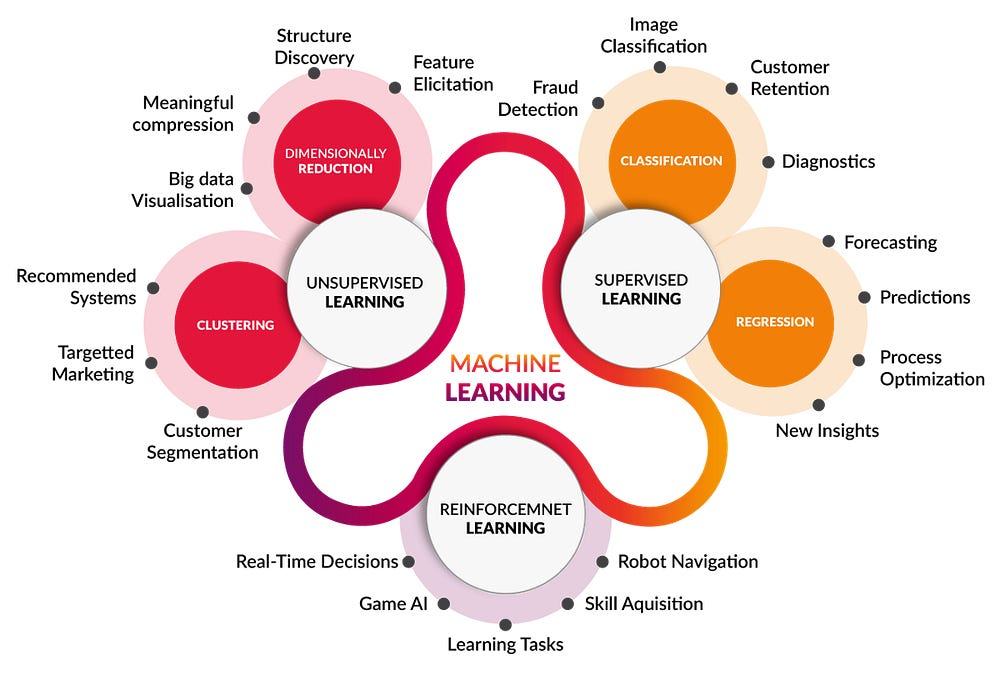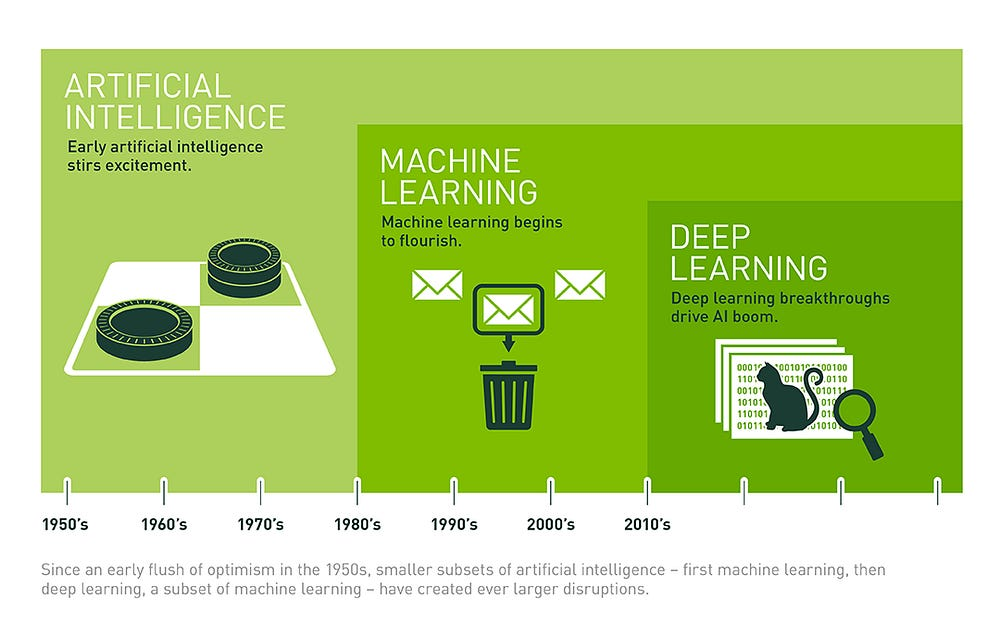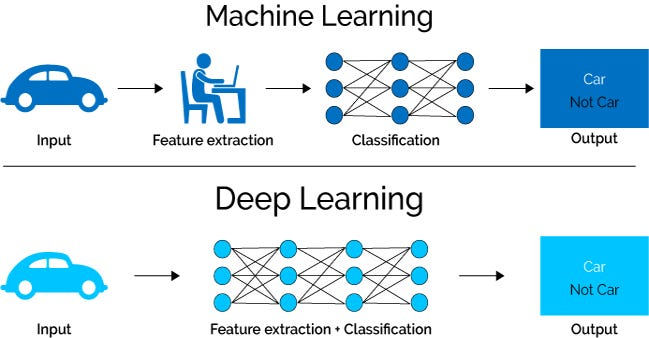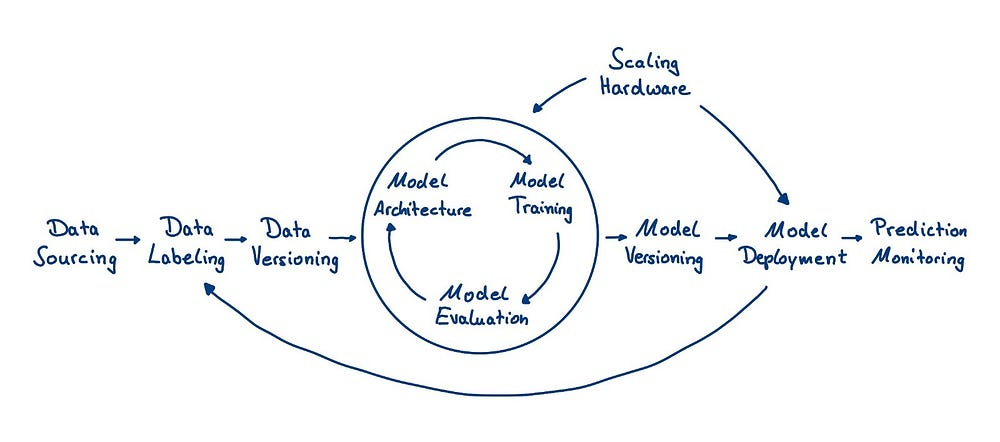# Deep Learning 101 for Dummies like Me

Source: Deep Learning on MediumImage source: http://www.cognub.com/index.php/cognitive-platform/

Currently, Artificial Intelligence is advancing at a great pace and deep learning is one of the biggest contributors to that. So, in the 2nd post of the 101 for Dummies like Me series, I’ll take you through the basics of deep learning. You can find the 1st post here where we talked about the basics of PyTorch, which was inspired by Intro to Deep Learning with PyTorch from Udacity. Some of the images in this post are taken from Udacity Deep Learning Nanodegree which is a great starting point for beginners. In the last years, applications of Deep Learning made huge advancements in many domains arousing astonishment in people that didn’t expect the technology and world to change so fast. Refer below image for a uber level comparison between AI vs. ML vs. DLImage source: https://www.datasciencecentral.com/profiles/blogs/artificial-intelligence-vs-machine-learning-vs-deep-learning

The hype started in March 2016 when Lee Sedol, the 18-time world champion was beaten 4 to 1 by super-computer AlphaGo. This match had a huge influence on the Go community as AlphaGo invented completely new moves which made people try to understand, reproduce them and created a totally new perspective on how to play the game. But that’s not over, in 2017 DeepMind introduced AlphaGo Zero. The newer version of an already unbeatable machine was able to learn everything without any starting data or human help. All that with computational power 4 times less than it’s predecessor!

### What is Deep Learning all about?

Deep learning is a branch of machine learning based on a set of algorithms that attempt to model high-level abstractions in data inspired by the structure and function of the brain called artificial neural networks.

In a simple case, consider the image on your left, where you have some sets of neurons: The leftmost layer of the network is called the input layer(L1), and the rightmost layer the output layer(L3) (which, in this example, has only one node). The middle layer of nodes is called the hidden layer(L2) because its values are not observed in the training set. We also say that our example neural network has 3 input units (not counting the bias unit), 3 hidden units, and 1 output unit. Similarly, if it’s a deep network, there are many layers between the input and output (and the layers are not made of neurons but it can help to think of it that way), allowing the algorithm to use multiple processing layers, composed of multiple linear and non-linear transformations.

Sidenote from Biology: Like our human brain has millions of neurons in a hierarchy and Network of neurons which are interconnected with each other via Axons and passes Electrical signals from one layer to another called synapses. This is how we humans learn things. Whenever we see, hear, feel and think something a synapse(electrical impulse) is fired from one neuron to another in the hierarchy which enables us to learn, remember and memorize things in our daily life since the day we were born.

A neural network having more than one hidden layer is generally referred to as a Deep Neural Network.

### How Deep Learning differ from Traditional Machine Learning?Image Source: http://aimagnifi.com/blog/index.php/2017/10/13/what-is-the-difference-between-machine-learning-and-deep-learning/

One of the major differences between machine learning and deep learning model is on the feature extraction area. Feature extraction is done by human in machine learning whereas deep learning model figures out by itself.

Deep learning models tend to perform well with the amount of data whereas old machine learning models stop improving after a saturation point.

Machine learning algorithms and deep learning algorithms have different problem-solving approaches, in one hand a machine learning algorithm breaks the problem into different levels where, at each level, the problem is solved and then the solution of each level is combined to form the solution of an entire problem while in deep learning the problem is solved end-to-end as a whole.

Machine learning algorithms interpret crisp rules while deep learning does not i.e. result interpretation is more appropriate in machine learning while deep learning lacks this ideality.

In general, the training time of deep learning algorithms is high due to the presence of so many parameters in the deep learning algorithms whereas machine learning comparatively takes lesser time in the training procedure. This is then reversed for the testing time. The testing time for machine learning is higher than deep learning.

### What are activation functions all about?

Activation functions are really important for an Artificial Neural Network to learn and make sense of something really complicated and Non-linear complex functional mappings between the inputs and response variable. They introduce non-linear properties to our Network. Their main purpose is to convert an input signal of a node in an A-NN to an output signal. That output signal now is used as an input in the next layer in the stack.

One of the simplest activation functions is the Heaviside step function. This function returns a 0 if the linear combination is less than 0. It returns a 1 if the linear combination is positive or equal to zero.

Specifically, in A-NN we do the sum of products of inputs(X) and their corresponding Weights(W) and apply an Activation function f(x) to it to get the output of that layer and feed it as an input to the next layer. Other activation functions you’ll see are the logistic (often called the sigmoid), tanh, and softmax functions.

### What is the error and loss function?

In most learning networks, the error is calculated as the difference between the actual output and the predicted output.

The function that is used to compute this error is known as Loss Function(J). Different loss functions will give different errors for the same prediction, and thus have a considerable effect on the performance of the model. One of the most widely used loss function is mean square error(MSE), which calculates the square of the difference between actual value and the predicted value. Different loss functions are used to deal with different type of tasks, i.e. regression and classification. Thus, loss functions are helpful to train a neural network. Given an input and a target, they calculate the loss, i.e difference between output and the target variable. Loss functions fall under four major category:

• Regressive loss functions which are used in case of regressive problems, that is when the target variable is continuous. Some examples are: Mean Square Error, Absolute Error & Smooth Absolute Error
• Classification loss functions used when the target variable y, is a binary variable, 1 for true and -1 for false. Some examples are: Binary Cross Entropy, Negative Log Likelihood, Margin Classifier & Soft Margin Classifier
• Embedding loss functions which deal with problems where we have to measure whether two inputs are similar or dissimilar. Some examples are:
1. L1 Hinge Error- Calculates the L1 distance between two inputs.
2. Cosine Error- Cosine distance between two inputs.

### How did Gradient Descent come into play?

“A gradient measures how much the output of a function changes if you change the inputs a little bit.” — Lex Fridman (MIT)

Gradient descent is an optimization algorithm used to find the values of parameters (coefficients) of a function (f) that minimizes a cost function (cost). Gradient descent is best used when the parameters cannot be calculated analytically (e.g. using linear algebra) and must be searched for by an optimization algorithm. Gradient descent is used to find the minimum error by minimizing a “cost” function.

Stochastic Gradient Descent performs a parameter update for each training example, unlike normal Gradient Descent which performs only one update. Thus it is much faster. Gradient Decent algorithms can further be improved by tuning important parameters like momentum(which determines the velocity with which learning rate has to be increased as we approach the minima), learning rate(a hyper-parameter that controls how much we are adjusting the weights of our network with respect the loss gradient) etc.

### The lifecycle of a typical Deep learning application?

The lifecycle of a typical (supervised) deep learning application consists of different steps, starting from raw data and ending with predictions in the wild.Image Source: https://medium.com/luminovo/the-deep-learning-toolset-an-overview-b71756016c06

Note: The flowchart already hints at the circular nature of the typical deep learning workflow. In fact, treating the feedback loop between deployed models and new labels (often called human-in-the-loop) as a first-class citizen in your deep learning workflow can be one of the most important success factors for many applications. In real-life deep learning work, things are often more complicated than the flowchart suggests. You will find yourself jumping over steps (e.g. when you are working with a pre-labeled dataset), going back several steps (model performance isn’t accurate enough and you need to source more data) or go back and forth in crazy loops (Architecture => Training => Evaluation => Training => Evaluation => Architecture).

### The deepest problem with deep learning

“Realistically, deep learning is only part of the larger challenge of building intelligent machines. Such techniques lack ways of representing causal relationships (such as between diseases and their symptoms), and are likely to face challenges in acquiring abstract ideas like “sibling” or “identical to.” They have no obvious ways of performing logical inferences, and they are also still a long way from integrating abstract knowledge, such as information about what objects are, what they are for, and how they are typically used. The most powerful A.I. systems … use techniques like deep learning as just one element in a very complicated ensemble of techniques, ranging from the statistical technique of Bayesian inference to deductive reasoning.”
Reference: Gary Marcus (AI Researcher, NYU)